Courses

# Flow Through Open Channels - 2

## 10 Questions MCQ Test Mock Test Series for Civil Engineering (CE) GATE 2020 | Flow Through Open Channels - 2

Description
This mock test of Flow Through Open Channels - 2 for Civil Engineering (CE) helps you for every Civil Engineering (CE) entrance exam. This contains 10 Multiple Choice Questions for Civil Engineering (CE) Flow Through Open Channels - 2 (mcq) to study with solutions a complete question bank. The solved questions answers in this Flow Through Open Channels - 2 quiz give you a good mix of easy questions and tough questions. Civil Engineering (CE) students definitely take this Flow Through Open Channels - 2 exercise for a better result in the exam. You can find other Flow Through Open Channels - 2 extra questions, long questions & short questions for Civil Engineering (CE) on EduRev as well by searching above.
QUESTION: 1

### A right-angled triangular channel symmetrical in section about the vertical carries a discharge of 5 m3/s with a velocity of 1.25 m/s. What is the approximate value of the Froude number of the flow?

Solution:

We know that,
Q = AV
⇒ 5 = A x 1.25 ⇒ A = 4 m2
For a symmetrical right angled triangular channel,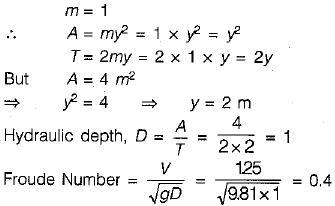QUESTION: 2

### A rectangular channel is 5 m wide and 3 m deep. Water is flowing in the channel for a depth of 2.5 m. The hydraulic radius is

Solution: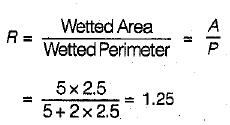QUESTION: 3

### Flow depths across a sluice gate are 2.0 m and 0.5 m. What is the discharge (per metre width)?

Solution: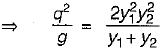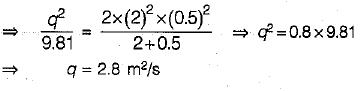QUESTION: 4

Given that
S0 = slope of the channel bottom
Se = slope of the energy line
F = Froude number
the equation of gradually varied flow is expressed as

Solution:
QUESTION: 5

A rectangular channel is 6 m wide and discharges 30 m3s-1. The upstream depth is 2.0 m, acceleration due to gravity is 10 ms-2. Then, what is the specific energy (approximate)?

Solution:

We know that,
Q = AV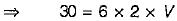⇒  \/ = 2.5 m/s
The specific energy may be given as,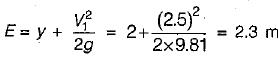QUESTION: 6

Most efficient channel section is

Solution:
QUESTION: 7

For a smooth hump in a subcritical flow to function as a broad crested weir, the height ΔZ of the hump above the bed must satisfy which one of the following?
(E1 = Specific head upstream of the hump, Ec = Specific head at the critical" depth yc) (Neglect friction effects)

Solution:

If the flow is subcritical, then after the introduction of hump the specific energy will decrease by ΔZ (height of hump).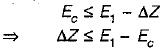QUESTION: 8

A hydraulic jump occurs at the top of a spillway. The depth before jump is 0.2 m. The sequent depth is 3.2 m. What is the energy dissipated in m (approximate)?

Solution:

The energy dissipation is given by,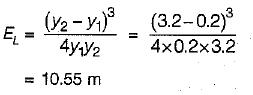QUESTION: 9

What is the normal depth in a wide rectangular channel carrying 0.5 m2/s discharge at a bed slope of 0.0004 and Manning’s n = 0.01?

Solution:

For a wide rectangular channel, the hydraulic radius (F) is approximately equal to the depth of flow. By Manning’s equation, we have,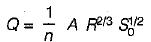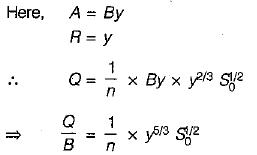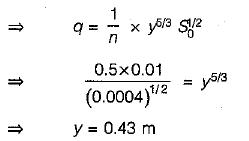QUESTION: 10

Match List-I (Flow section type) with List-ll (Critical discharge is proportional to) where y is the depth of flow-and select the correct answer using the codes given below the lists: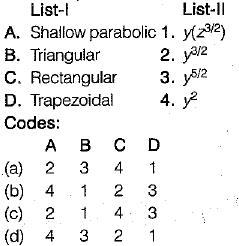Solution: